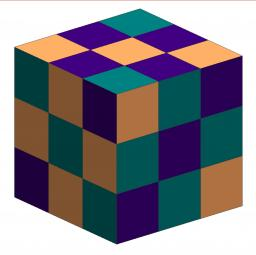# Cube volume

The cube has a surface of 384 cm2. Calculate its volume.

V =  512 cm3

### Step-by-step explanation:Did you find an error or inaccuracy? Feel free to write us. Thank you!Tips to related online calculators
Tip: Our volume units converter will help you with the conversion of volume units.

## Related math problems and questions:

• Wall diagonalCalculate the length of wall diagonal of the cube whose surface is 384 cm square.
• Cube 1-2-3Calculate the volume and surface area of the cube ABCDEFGH if: a) /AB/ = 4 cm b) perimeter of wall ABCD is 22 cm c) the sum of the lengths of all edges of the cube is 30 cm.
• Cube surface and volumeThe surface of the cube is 500 cm2, how much cm3 will be its volume?
• Cube diagonalsDetermine the volume and surface area of the cube if you know the length of the body diagonal u = 216 cm.
• Surface areaThe volume of a cone is 1000 cm3 and the content area of the axis cut is 100 cm2. Calculate the surface area of the cone.
• Cube surfce2volumeCalculate the volume of the cube if its surface is 150 cm2.
• Surface and volume - cubeFind the surface and volume of a cube whose wall diagonal is 5 cm long.
• Volume and areaWhat is the volume of a cube which has an area of 361 cm2?
• Area to volumeIf the surface area of a cube is 486, find its volume.
• Magnified cubeIf the lengths of the cube's edges are extended by 5 cm, its volume will increase by 485 cm3. Determine the surface of both the original and the magnified cube.
• The cubeThe cube has a surface area of 486 m ^ 2. Calculate its volume.
• Cube 6Volume of the cube is 216 cm3, calculate its surface area.
• Body diagonal - cubeCalculate the surface and cube volume with body diagonal 15 cm long.
• Cuboid wallsCalculate the cuboid volume if its different walls have an area of 195cm², 135cm², and 117cm².
• Equilateral cylinderEquilateral cylinder (height = base diameter; h = 2r) has a volume of V = 199 cm3 . Calculate the surface area of the cylinder.
• Area of a cubeCalculate the surface area of a cube if its volume is equal to 729 cubic meters.
• Cube diagonalsThe cube has a wall area of 81 cm square. Calculate the length of its edge, wall, and body diagonal.